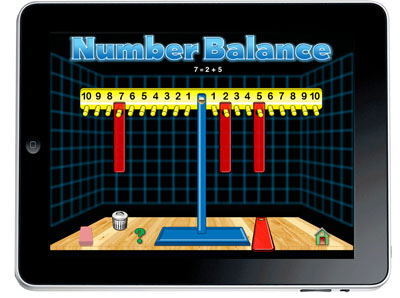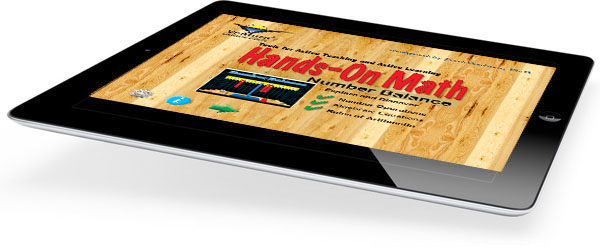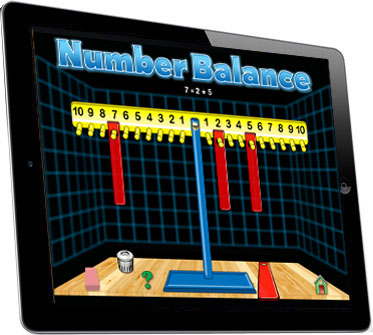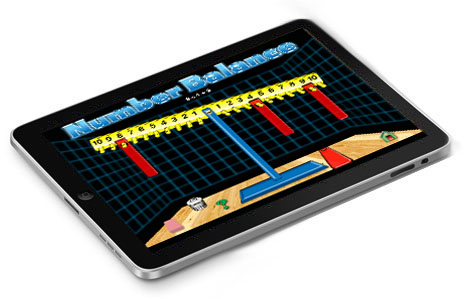Work with Equalities and Inequalities using this Interactive App!Hands-On Math Number Balance provides an interactive learning enviroment for learning and enhancing addition and multiplication skills, and problem solving abilities. It is a perfect classroom tool for core mathematics instruction and enrichment. While using this app students will use mathematical thinking skills to solve randomly generated problems. Students will find the challenges solvable through interaction. The skills learned are relevant to the elementary mathematics curriculum. Encourage your students to try our Number Balance, and as they do you will see them get better at many basic math skills.

Number Balance offers 8 levels of difficulty. Younger learners will benefit from the settings where the challenge is to find the sum or product by balancing the scale. For older students teachers may want to use some of the options where the challenge is to find a missing addend, add a set of 3 or 4 numbers or find products.

Levels:

• Level 1: Numbers and Operations - Comparing Whole Numbers
• Level 2: Addition Skills - Basic Addition Facts - Whole Numbers from 1 to 10
• Level 3: Addition Skills - Basic Addition Facts - Whole Numbers from 1 to 20
• Level 4: Addition Skills - Focus on Problem Solving - Whole Numbers from 1 to 30
• Level 5: Multiplication Skills - Multiplication Facts - Whole Numbers from 1 to 5
• Level 6: Multiplication Skills - Multiplication Facts - Whole Numbers from 1 to 10
• Level 7: Multiplication Skills - Focus on Patterns - Whole Numbers from 1 to 10
• Level 8: Addition Skills - Three or More Addends - Whole Numbers from 1 to 10
 Hands-On Math Number BalanceProblem Solving and Skill Building Learning addition and multiplication facts can be fun. Number Balance goes beyond drill and practice and presents addition and multiplication practice activities in a way that student can through discovery. At the beginning levels bars are randomly placed on the balance and the student is challenged to find a way to make the balance level. Math makes sense! Videos: Hands-On Math Number Balance creates a virtual mathematical playground right on your iPad. It simulates the use of a popular math manipulative (a number balance) and combines it with a creative problem solving activity. It is perfect for elementary and middle school classrooms. Enrich your mathematics curriculum with Hands-On Math Number Balance. Visualization is one of the best ways to help students explore and discover mathematics. Students who use Number Balance will develop an intuitive understanding of equivalency. In addition students will use mathematical reasoning to solve each challenging questions which are randomly generated at eight different levels. Using Hands-On Math Number Balance students can develop the following important mathematical concepts: Addition Facts Multiplication Facts Comparing Whole Numbers Equations and Inequalities Mathematical ReasoningWe recommend that you open the Instructor's Guide PDF from your iPad in a new browser page and save the document in your iBooks app. Addition Concepts and Skills Multiplication Concepts and Skills Problem Solving and Missing Terms Special Features Using Math Skills to Solve Problems with the Number Balance Here's a quick demo to show how much fun it is to use Hands-On Math Number Balance. (Click to see movie.) Features: Interactive Number Balance for Interaction and Discovery. Supports Common Core Objectives Focuses on Problem Solving Strategies. Problem Solving Activities which Can Be Solved Using Virtual Manipulatives. The Hands-On Math Series of Apps Helps Build Fundamental Math Concepts. On/Off Options and Controls for Various Features.\$2.99 Now Available at the Apple iTunes Store.
 Number Balance Number Balance is a fun way to develop addition and multiplication skills. Using simple gestures students move and place bars on the number balance. Student interaction is instantly represented as mathematical expressions. Possible Topics: Addition Facts Multiplication Facts Problem Solving Comparisons ( <, >, = ) Mathematical Reasoning Equality and InequalityNumber Balance Functions By dragging an bar onto the balance, the student defines mathematical expressions. Depending on the level setting the student may receive instantaneous feedback regarding the correctness of the bar placement. Select Levels by Tapping the Settings Icon Choose Addition or Multiplication Determine Range of Number Optional Sounds and Speech FunctionsExplore and Document Activities at a variety of skill levels are also provided. Students are encouraged to document their discoveries in a journal. A comprehensive teacher's guide provides lesson ideas at a variety of levels. Perfect for Small Group instruction Environmentally Friendly Learning - (no need to photocopy activity pages for each student) A Student-Centered Approach to LearningHands-On Math: Number Balance is now available at the Apple iTunes Store.